Newton's rings are known as one of the greatest findings of Isaac Newton. The original observation was first made with a wedge-shaped air-gap between the surfaces of two prisms, but he also found the same phenomenon happening when the convex surface of a lens superimposes on a flat glass plate. In fact, Newton tried to explain this phenomenon as a part of his theory; the light consistes of little particles. However, later on, Newton's Rings became a clear evedence of the wave nature of light.

• Reflection

The light hits on the substance and reflects.
For example, the light that travels in the air hits on the glass, and reflects. Here, the incident angle and the reflection angle are the same.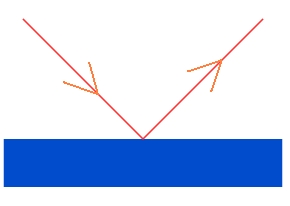More precisely, the light can hit on the top surface and reflects (1), or the light first refracts and goes into the glass, and reflects at the bottom, and then refracts out (2).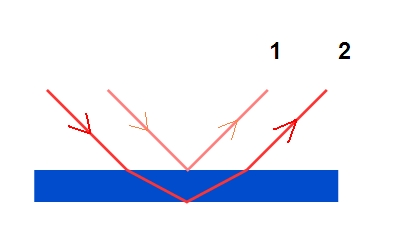Sometimes, when the light are perpendicular to the substance, the light transmits without bending.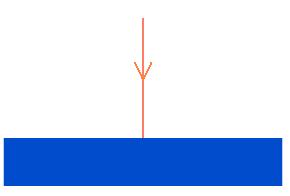Then, the light keeps travelling in the new substance, and eventually hits at the bottom of the material, and transmits again.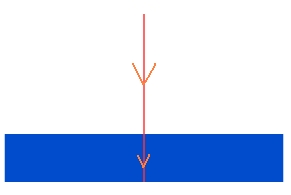• Newton's Rings Apparatus

In addition to the flat glass, a spherical glass is placed on that with its convex side against the flat one.If the lights reach the flat surface of the spherical glass, the lights first transmit, and then either reflect on the spherical surface or transmit and hit on the flat surface.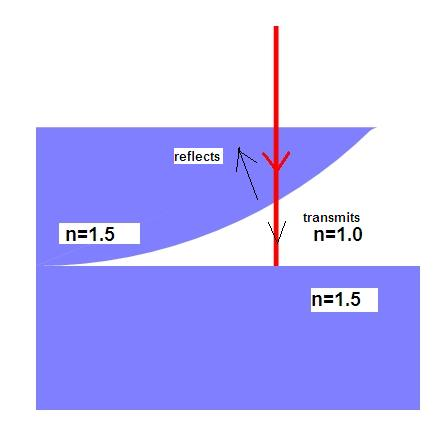Next, the conditions of the reflection on the spherical surface and on the flat surface are different. Here is the key point of explaining the wave nature of light. Since the light travels as a wave, the phase change occurs under a certain setting.

Phase Change Rules due to Reflection

• Reflection off larger index of refraction: phase change a half of the wavelength.(A)

• Reflection off lower index of refraction: no phase change.(B)

• Transmitting: no phase changes.(C)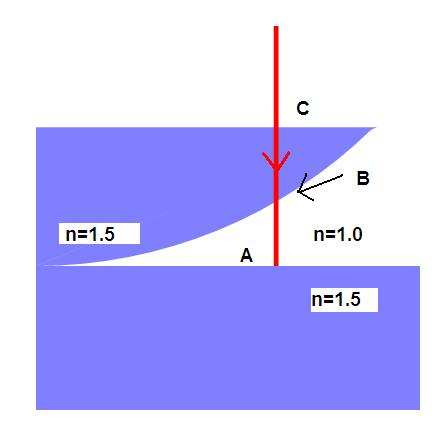• Interference
So, where do the lights with different phases go? Do they travel separately or go together?
Indeed, the light that reflects on the spherical surface and the light reflects on the flat surface meet and interfere.
Let's start from observing the waves.
Since the light travels as a wave, the simplest way to express the light is drawing a sine curve.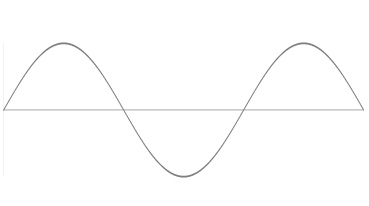When the two lights meet with the same phase, two waves interfere, and create a bigger wave. It occurs when two waves have exactly the same phase, and when the phases are 2ndifferent (i.e. n times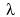equivalent. where n=0,1,2,...)On the other hand, if the two lights meet with different phases, they eliminate each other. Thus, the amplitude of the resultant wave becomes smaller.
Especially, when the phase difference is n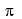(n times 1/2equivalent, where n=0,1,3,5,...), two waves completely eliminate each other.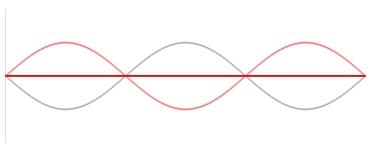Now, we call the distance between two glasses "d".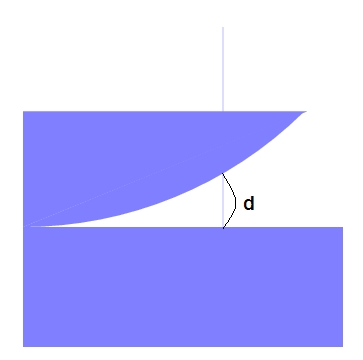Then, the light that transmits and reflects on the flat surface travels 2d longer than the light that reflects on the spherical surface.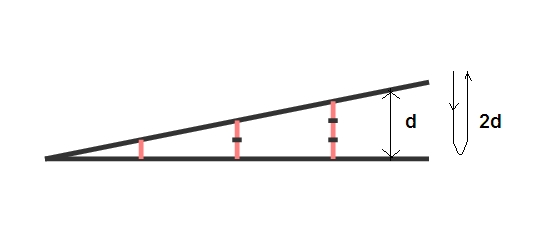Therefore, the total phase change (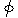) is 2d + phase change caused by the reflection on the flat surface. When this distance 2d equals one wavelength: (phase change) =+ 1/2The two lights eliminate each other, and we see dark rings.(i.e. 2d= 1/2()(2m) where m=0,1, 2,...) As well as the dark ones, the bright rings appears when 2d equals 1/2 wavelength such as 1/2(2m+1). (where m=0,1,2,...)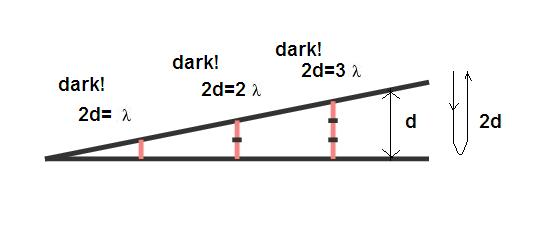In sum,

dark rings:2d=(1/2)(2m)

bright rings:2d=(1/2)(2m+1)

where m= 0,1,2,3,...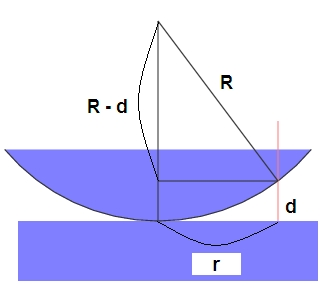Let's express these equations with radius(r) where the distance from the centre to the place of the fringes.
Suppose the radius(R) of the spherical glass is very very large. Then, the distance(d) becomes very very small.

(R-d)2 + r2 = R2

Since the distance is very very small, R2 - 2dR + d2 + r2 = R2,
d2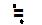= 0.
Thus,
2 dr2 / R

dark rings: 2 d = r2 / R = (1/2)(2m)

bright rings: 2 d = r2 / R = (1/2)(2m+1)

where m= 0,1,2,3,...

Finally, we obtain
DARK RINGS:
rdark =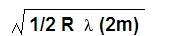BRIGHT RINGS:
rbright =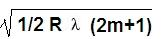where m=0,1,2,...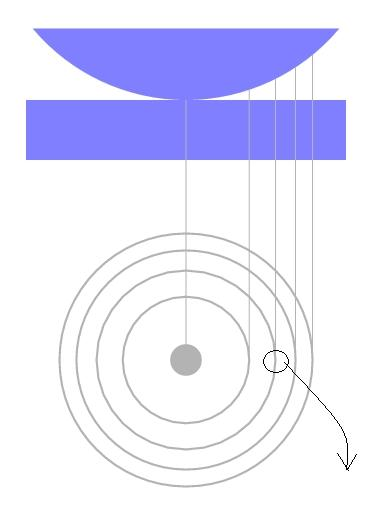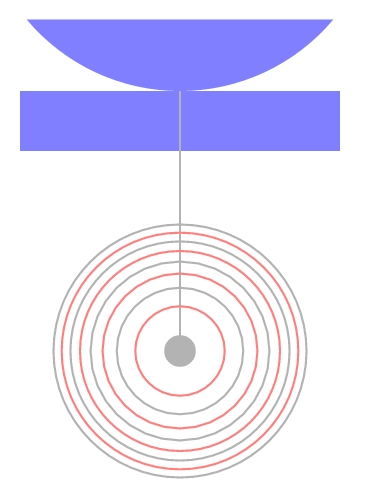Also, around the crests, the intensity becomes minimum due to the interference, (in this case, complete elimination happened.).
The darkness becomes gradually lighter as it goes further from the crests."Newton's rings" looks like this!!!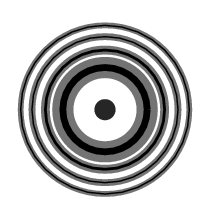• Monochromatic Lights vs White Light

The monochromatic lights such as red, green, violet lights have different wave lengths. How do the Newton's rings differ by this?

• Red light
The wavelength is 780 nm.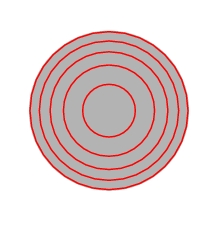• Green light
The wavelength is 550 nm.• Violet Light
The wavelength is 380 nm.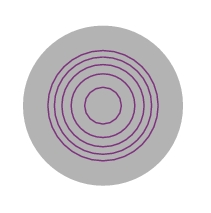The rings' radii are bigger in longer waves and smaller in shorter waves. Thus, if the white light goes through the Newton's rings apparatus, the each ray is separated, and reflects in different ways. As a result, the violet makes inner layers of the bright rings, and red makes outer layers on every bright part.
However, as the radius increases, many lights interfere and are mixed together. Then, the colorful bands can no longer be seen clearly.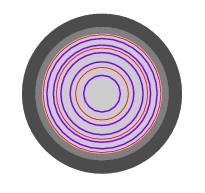References:
Opticks: Isaac Newton. Fourth Edition. 1979. Dover Publications.New York.
Light: R. W.Ditchburn. Volume One. 1976. Academic Press. London.